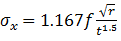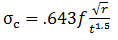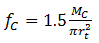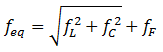top of page

#### Local Stress Calculation Based on Kellogg’s Model

For local stress calculation in a discontinuity area, depending on loading condition, a pipe stress engineer can use two models: Ring Model and Chocking Model. For example in analysis of local stress in saddles, the Ring Model in which tangential forces and circumferential moments develop circumferential membrane stresses and circumferential bending stresses is used. Whereas Kellogg’s method is based on chocking model in which local stresses including circumferential membrane stress and longitudinal bending stress are developed because a radial load tends to chock the pipe shell. Using this method usually put engineer in a conservative area because at junction of a trunnion and pipe, the pipe shell is not fully chocked.

Firstly the method limitations are described and then the concept of the method is explained in details. Finally the Kellogg’s method which has been developed by Kellogg’s company and used by many companies around the world is reviewed briefly. So piping stress engineers may decide how to use the chocking model to ensure the design is safe.

1- Assumptions and limitations

• Shear forces are ignored.

• Torsional moment is ignored.

• Both in-plane and out-plane bending moments are included.

2- Developing of basic formula

Referring to Chocking Model, figure 1, two types of local stresses significantly exist: Longitudinal bending stresses resulting from a bending moment which tends to bend the pipe shell in the discontinuity area and circumferential membrane stresses resulting from a circumferential force which is developed due to material resistance against radial deformation caused by the uniform external radial force (Figure 2).Figure 1: Chocking ModelFigure 2: Significant local stresses developed in a chocking model

Longitudinal bending stress was calculated in Choking Model as :(1)

Circumferential membrane stress was calculated in Choking Model as :(2)

2-1-f Calculation:

In this model it is assumed that support loads are applied uniformly on the attachment area as follows:

#### 2-1-1-Equivalent uniform force per length due to axial load(3)

2-1-2-Equivalent uniform force per length due to axial load

(4)(5)

The stress resulting from an axial force is not uniformly distributed in such a way that its maximum value occurs in the edge of attached area. So a factor of 1.5 is considered in Kellogg’s method. Therefore(6)

The distribution pattern of external circumferential bending moment,     , is different from the normal one in such a way the maximum stress occurs in different place not at the edge of attachment area. So similar to axial force, a factor of 1.5 should be considered in the calculation:(7)

The equivalent of f can be calculated by the following formula:(8)

In case of using pad, total pipe wall and pad thickness may be used.

The two local stresses, equations (1) and (2), can be calculated by substituting of      in the equations.3-Formula presented by Kellogg

Referring to “Kellogg Design Manual” only one formula for longitudinal and circumferential local stresses calculation is used:                . The only parameter which makes a longitudinal stress distinct from a circumferential one is f. There are two values introduced by the manual.              represent loads producing maximum local stresses in longitudinal and circumferential direction respectively with consider appropriate factors mentioned in table A of . If sum of pressure and Kellogg’s local stresses meet the allowable stresses which are introduced in table B of , the system is accepted by this method. The method has been successfully used for piping industries for many years. If an engineer is willing to use the Kellogg’s method, the design manual, , should be read thoroughly. There are some notes in the manual which should be noticed. For example, in expansion load case, a factor of 1.5 is not considered in calculation of                       .

As a result, an engineer may refer to equations (1) and (2) which are based on chocking model to calculate longitudinal bending local stress and circumferential membrane local stress with a factor of 1.5. These local stresses may be combined with other membrane and bending stresses resulting from primary loads (such as internal pressure and sustained loads) and secondary loads (such as thermal loads) in a proper way which is presented by ASME BPVC section VIII Div2.

Stress engineers are accountable for designing a safe system. So this responsibility make an engineer use a trustable method such as finite element analysis for a critical system in lieu of Kellogg’s method or any other analytical method.bottom of page